#.

Bayesian Nonparametrics is a class of models with a potentially infinite number of parameters. High flexibility and expressive power of this approach enables better data modelling compared to parametric methods.

Bayesian Nonparametrics is used in problems where a dimension of interest grows with data, for example, in problems where the number of features is not fixed but allowed to vary as we observe more data. Another example is clustering where the number of clusters is automatically inferred from data.

Our team asked a data scientist, Vadim Smolyakov, to introduce us to Bayesian Nonparametric models. In this article, he describes the Dirichlet process along with associated models and links to their implementations.### Introduction: Dirichlet process K-means

Bayesian Nonparametrics are a class of models for which the number of parameters grows with data. A simple example is non-parametric K-means clustering . Instead of fixing the number of clusters K, we let data determine the best number of clusters. By letting the number of model parameters (cluster means and covariances) grow with data, we are better able to describe the data as well as generate new data given our model.

Of course, to avoid over-fitting, we penalize the number of clusters K via a regularization parameter which controls the rate at which new clusters are created. Thus, our new K-means objective becomes:

In the figure above, we can see the non-parametric clustering, aka Dirichlet-Process (DP) K-Means applied to the Iris dataset. The strength of regularization parameter lambda (right), controls the number of clusters created. Algorithmically, we create a new cluster, every time we discover that a point (x_i) is sufficiently far away from all the existing cluster means: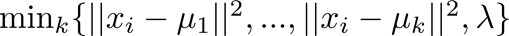The resulting update is an extension of the K-means assignment step: we reassign a point to the cluster corresponding to the closest mean or we start a new cluster if the squared Euclidean distance is greater than lambda. By creating new clusters for data points that are sufficiently far away from the existing clusters, we eliminate the need to specify the number of clusters K ahead of time.

Dirichlet process K-means eliminates the need for expensive cross-validation in which we sweep a range of values for K in order to find the optimum point in the objective function. For an implementation of the Dirichlet process K-means algorithm see the following github repo.

### Dirichlet process

The Dirichlet process (DP) is a stochastic process used in Bayesian nonparametric models . Each draw from a Dirichlet process is a discrete distribution. For a random distribution G to be distributed according to a DP, its finite dimensional marginal distributions have to be Dirichlet distributed. Let H be a distribution over theta and alpha be a positive real number.

We say that G is a Dirichlet process with base distribution H and concentration parameter alpha if for every finite measurable partition A1,…, Ar of theta we have: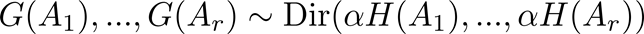Where Dir is a Dirichlet distribution defined as: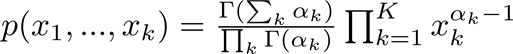The Dirichlet distribution can be visualized over a probability simplex as in the figure below. The arguments to the Dirichlet distribution (x1, x2, x3) can be interpreted as pseudo-counts.

For example, in the case of (x1, x2, x3) = (2, 2, 2) the Dirichlet distribution (left) has high probability near the middle, in comparison to the (2, 2, 10) case where it concentrates around one of the corners. In the case of (10, 10, 10) we have more observations, and the Dirichlet distribution concentrates more in the middle (since equal number of counts are observed in this case).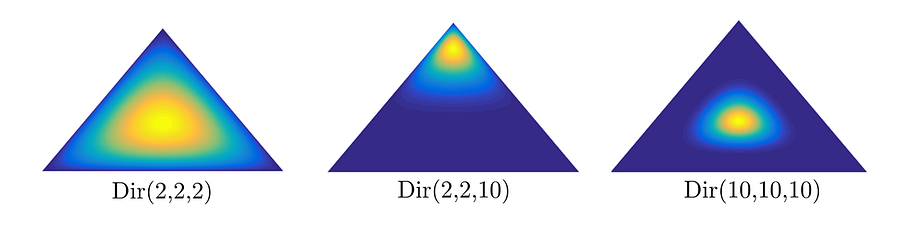The base distribution H is the mean of the DP: E[G(A)] = H(A), whereas the concentration parameter is the inverse variance: VAR[G(A)] = H(A)[1-H(A)] / (1+alpha). Thus, the larger the alpha, the smaller the variance and the DP will concentrate more of its mass around the mean as shown in the figure below .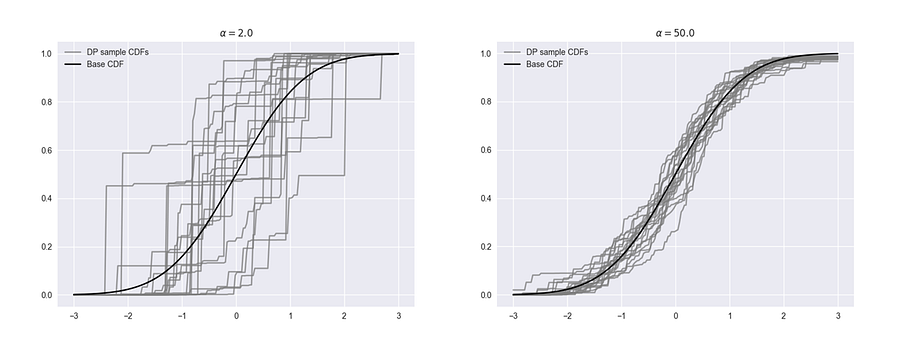### Stick-Breaking Construction

We have seen the utility of Bayesian Nonparametric models is in having a potentially infinite number of parameters. We also had a brief encounter with the Dirichlet process that exhibits a clustering property that makes it useful in mixture modeling where the number of components grows with data.

But how do we generate a mixture model with an infinite number of components?

The answer is a stick-breaking construction  that represents draws G from DP(alpha, H) as a weighted sum of atoms (or point masses). It is defined as follows: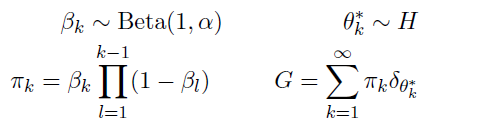The mixture model G consists of an infinite number of weights (pi_k) and mixture parameters (theta_k). The weights are generated by first sampling beta_k from Beta(1, alpha) distribution, where alpha is the concentration parameter and then computing pi_k as in the expression above, while mixture parameters theta_k are sampled from the base distribution H. We can visualize the stick-breaking construction as in the figure below:

Notice that we start with a stick of unit length (left) and in each iteration we break off a piece of length pi_k. The length of the piece that we break off is determined by the concentration parameter alpha. For alpha=5 (middle) the stick lengths are longer and as a result there are fewer significant mixture weights. For alpha=10 (right) the stick lengths are shorter and therefore we have more significant components.

Thus, alpha determines the rate of cluster growth in a non-parametric model. In fact, the number of clusters created is proportional to alpha x log(N) where N is the number of data points.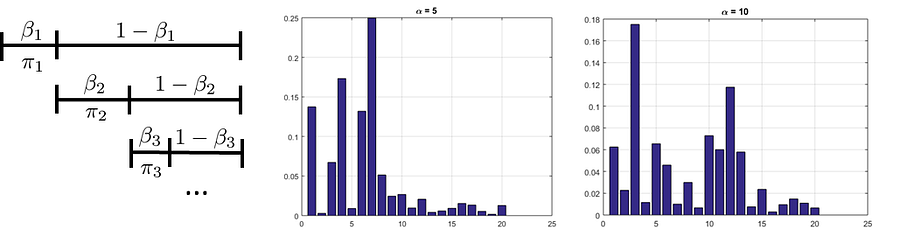### Dirichlet process Mixture Model (DPMM)

A Dirichlet process mixture model (DPMM) belongs to a class of infinite mixture models in which we do not impose any prior knowledge on the number of clusters K. DPMM models learn the number of clusters from the data using a nonparametric prior based on the Dirichlet process (DP). Automatic model selection leads to computational savings of cross validating the model for multiple values of K. Two equivalent graphical models for a DPMM are shown below: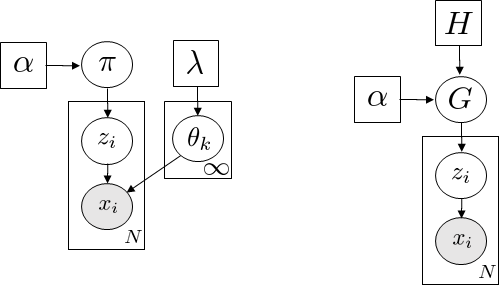Here, x_i are observed data points and with each x_i we associate a label z_i that assigns x_i to one of the K clusters. In the left model, the cluster parameters are represented by pi (mixture proportions) and theta (cluster means and covariances) with associated uninformative priors (alpha and lambda).

For ease of computation, conjugate priors are used such as a Dirichlet prior for mixture weights and Normal-Inverse-Wishart prior for a Gaussian component. In the right model, we have a DP representation of DPMM where the mixture distribution G is sampled from a DP (alpha, H) with concentration parameter alpha and base distribution H.

There are many algorithms for learning the Dirichlet process mixture models based on sampling or variational inference.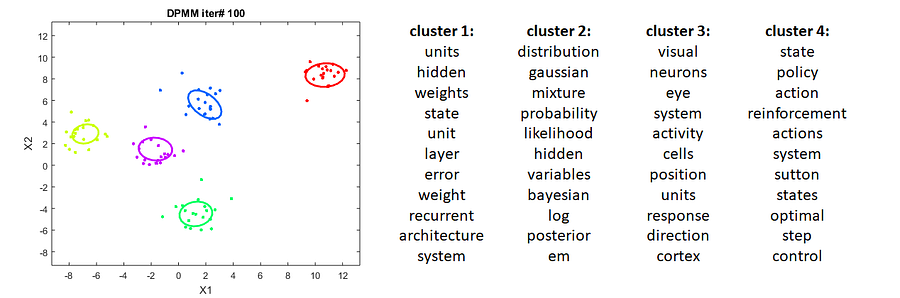The figure above shows DPMM clustering results for a Gaussian distribution (left) and Categorical distribution (right). On the left, we can see the ellipses (samples from posterior mixture distribution) of the DPMM after 100 Gibbs sampling iterations. The DPMM model initialized with 2 clusters and a concentration parameter alpha of 1, learned the true number of clusters K=5 and concentrated around cluster centers.

On the right, we can see the results of clusters of Categorical data, in this case a DPMM model was applied to a collection of NIPS articles. It was initialized with 2 clusters and a concentration parameter alpha of 10. After several Gibbs sampling iterations, it discovered over 20 clusters, with the first 4 shown in the figure. We can see that the word clusters have similar semantic meaning within each cluster and the cluster topics are different across clusters.

### Hierarchical Dirichlet process (HDP)

The hierarchical Dirichlet process (HDP) is an extension of DP that models problems involving groups of data especially when there are shared features among the groups. The power of hierarchical models comes from an assumption that the features among groups are drawn from a shared distribution rather than being completely independent. Thus, with hierarchical models we can learn features that are common to all groups in addition to the individual group parameters.

In HDP, each observation within a group is a draw from a mixture model and mixture components are shared between groups. In each group, the number of components is learned from data using a DP prior. The HDP graphical model is summarized in the figure below :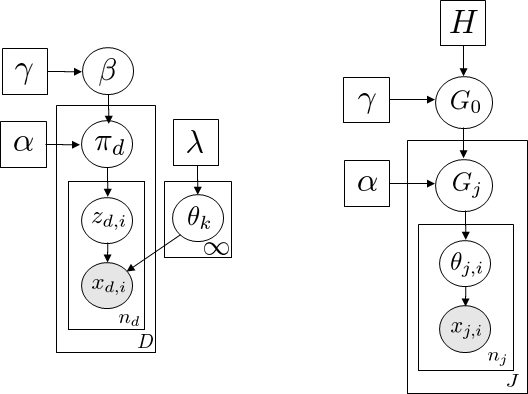Focusing on HDP formulation in the figure on the right, we can see that we have J groups where each group is sampled from a DP: Gj ~ DP(alpha, G0) and G0 represents shared parameters across all groups which in itself is modeled as a DP: G0 ~ DP(gamma, H). Thus, we have a hierarchical structure for describing our data.

There exists many ways for inferring the parameters of hierarchical Dirichlet processes. One popular approach that works well in practice and is widely used in the topic modelling community is an online variational inference algorithm  implemented in gensim.The figure above shows the first four topics (as a word cloud) for an online variational HDP algorithm used to fit a topic model on the 20newsgroups dataset. The dataset consists of 11,314 documents and over 100K unique tokens. Standard text pre-processing was used, including tokenization, stop-word removal, and stemming. A compressed dictionary of 4K words was constructed by filtering out tokens that appear in less than 5 documents and more than 50% of the corpus.

The top-level truncation was set to T=20 topics and the second level truncation was set to K=8 topics. The concentration parameters were chosen as gamma=1.0 at the top-level and alpha=0.1 at the group level to yield a broad range of shared topics that are concentrated at the group level. We can find topics about autos, politics, and for sale items that correspond to the target labels of the 20newsgroups dataset.

### HDP hidden Markov models

The hierarchical Dirichlet process (HDP) can be used to define a prior distribution on transition matrices over countably infinite state spaces. The HDP-HMM is known as an infinite hidden Markov model where the number of states is inferred automatically. The graphical model for HDP-HMM is shown below: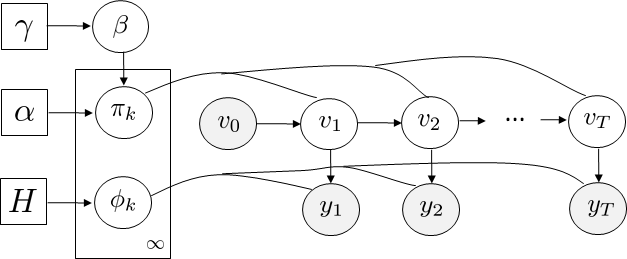In a nonparametric extension of HMM, we consider a set of DPs, one for each value of the current state. In addition, the DPs must be linked because we want the same set of next states to be reachable from each of the current states. This relates directly to HDP, where the atoms associated with state-conditional DPs are shared.

The HDP-HMM parameters can be described as follows: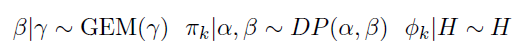Where the GEM notation is used to represent stick-breaking. One popular algorithm for computing the posterior distribution for infinite HMMs is called beam sampling and is described in .

### Dependent Dirichlet process (DDP)

In many applications, we are interested in modelling distributions that evolve over time as seen in temporal and spatial processes. The Dirichlet process assumes that observations are exchangeable and therefore the data points have no inherent ordering that influences their labelling. This assumption is invalid for modelling temporal and spatial processes in which the order of data points plays a critical role in creating meaningful clusters.

The dependent Dirichlet process (DDP), originally formulated by MacEachern, provides a nonparametric prior over evolving mixture models. A construction of the DDP built on the Poisson process  led to the development of the DDP mixture model as shown below: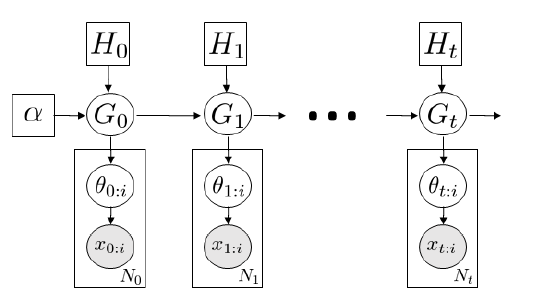In the graphical model above we see a temporal extension of the DP process in which a DP at time t depends on the DP at time t-1. This time-varying DP prior is capable of describing and generating dynamic clusters with means and covariances changing over time.

### Conclusion

In Bayesian Nonparametric models the number of parameters grows with data. This flexibility enables better modeling and generation of data. We focused on the Dirichlet process (DP) and key applications such as DP K-means (DP-means), Dirichlet process mixture models (DPMMs), hierarchical Dirichlet processes (HDPs) applied to topic models and HMMs, and dependent Dirichlet processes (DDPs) applied to time-varying mixtures.

We looked at how to construct nonparametric models using stick-breaking and examined some of the experimental results. To better understand the Bayesian Nonparametric model, I encourage you to read the literature mentioned in the references and experiment with the code linked throughout the article on challenging datasets!

### References

 B. Kulis and M. Jordan, “Revisiting k-means: New Algorithms via Bayesian Nonparametrics ”, ICML, 2012

 E. Sudderth, “Graphical Models for Visual Object Recognition and Tracking”, PhD Thesis (Chp 2.5), 2006

 A. Rochford, Dirichlet process Mixture Model in PyMC3

 J. Sethuraman, “A constructive definition of Dirichlet priors”, Statistica Sinica, 1994.

 Y. Teh, M. Jordan, M. Beal and D. Blei, “Hierarchical Dirichlet process”, JASA, 2006

 C. Wang, J. Paisley, and D. Blei, “Online Variational Inference for the Hierarchical Dirichlet process”, JMLR, 2011.

 J. Van Gael, Y. Saatci, Y. Teh and Z. Ghahramani, “Beam Sampling for the infinite Hidden Markov Model”, ICML 2008

 D. Lin, W. Grimson and J. W. Fisher III, “Construction of Dependent Dirichlet processes based on compound Poisson processes”, NIPS 2010

Views: 7851

Comment